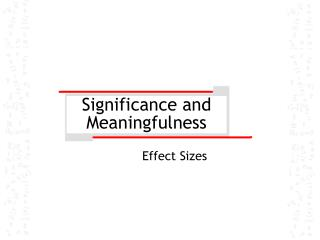Download PresentationSignificance and MeaningfulnessSignificance and Meaningfulness - PowerPoint PPT Presentation

Download PresentationSignificance and Meaningfulness
An Image/Link below is provided (as is) to download presentation

Download Policy: Content on the Website is provided to you AS IS for your information and personal use and may not be sold / licensed / shared on other websites without getting consent from its author. While downloading, if for some reason you are not able to download a presentation, the publisher may have deleted the file from their server.

- - - - - - - - - - - - - - - - - - - - - - - - - - - E N D - - - - - - - - - - - - - - - - - - - - - - - - - - -
Presentation Transcript

1. Significance and Meaningfulness Effect Sizes

2. Significance vs. meaningfulness • Is your significant difference a real difference?

3. Significance vs. meaningfulness • Is your significant difference a real difference?

4. Significance vs. meaningfulness • Statistical Power

5. Significance vs. meaningfulness • Statistical Power • Smaller difference between means reduces power • Larger SEM reduces power

6. Significance vs. meaningfulness • Statistical Power • Smaller  reduces power

7. Significance vs. meaningfulness • As sample size increases, likelihood of significant difference increases The fact that this sample size is buried down here in the denominator of the test statistic means that as n  , p  0. So if your sample is big enough, it will generate significant results

8. Significance vs. meaningfulness • As sample size increases, likelihood of significant difference increases • So statistical difference does not always mean important difference • What to do about this? • Calculate a measure of the difference that is standardized to be expressed in terms of the variability in the 2 samples • = EFFECT SIZE

9. Significance vs. meaningfulness • EFFECT SIZE - FORMULA

10. Significance vs. meaningfulness • EFFECT SIZE – from SPSS • Using appendix B data set 2, and submitting DV salary to test of difference across gender, gives the following output (squashed here to fit): T-Test

11. Significance vs. meaningfulness • EFFECT SIZE – from SPSS Mean difference to use T-Test SD’s to pool

12. Significance vs. meaningfulness • EFFECT SIZE – from SPSS So…

13. Significance vs. meaningfulness • EFFECT SIZE – from SPSS Substituting…

14. Significance vs. meaningfulness • EFFECT SIZE – from SPSS Calculating…

15. Significance vs. meaningfulness • From Cohen, 1988: • d = .20 is small • d = .50 is moderate • d = .80 is large • So our effect size of .25 is small, and concurs on this occasion with the insignificant result • The finding is both insignificant and small • (a pathetic, measly, piddling little difference of no consequence whatsoever – trivial and beneath us)

16. Statistical Power Maximizing the likelihood of significance

17. Statistical Power • The likelihood of getting a significant relationship when you should (i.e. when there is a relationship in reality) • Recall from truth table, power = 1 -  ( = type II error)

18. Factors Affecting Statistical Power The big ones: • Effect size (bit obvious) • Select samples such that difference between them is maximized • Sample size • Most important: as n increases, SEM decreases, and test statistic then increases

19. Factors Affecting Statistical Power The others: • Level of significance • Smaller , less power • Larger , more power • 1-tailed vs. 2-tailed tests • With good a priori info (i.e. research literature), selecting 1-tailed test increases power • Dependent samples • Correlation between samples reduces standard error, and thus increases test statistic

20. Calculating sample size a priori • Specify effect size • Set desired level of power • Enter values for effect size and power in appropriate table, and generate desired sample size: • Applet for calculating sample size based on above: http://www.stat.uiowa.edu/~rlenth/Power/ • Applets for seeing power acting (and interacting) with sample size, effect size, etc… http://statman.stat.sc.edu/~west/applets/power.html http://acad.cgu.edu/wise/power/powerapplet1.html http://www.stat.sc.edu/%7Eogden/javahtml/power/power.html